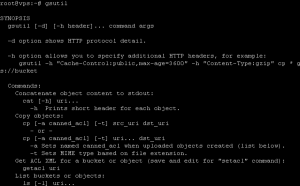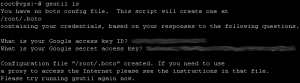• Chrome Frame for IE
• Firefox 3.6+
• Safari 4.0+

GSUtil ToolGSUtil Tool是更强大的命令行管理工具，可以安装在Linux和Mac OS上，也可以通过Cygwin安装在Windows上。第一次运行GSUtil Tool时候需要进行简单的配置，如果还没有手工配置直接输入命令就会出现提示，输入授权ID和访问KEY重新执行刚才的命令就可以了。```gsutil mb gs://cats gs://dogs
```

```gsutil cp *.jpg gs://dogs
```

```gsutil ls
gsutil ls gs://dogs
```

```gsutil mv gs://dogs/*.jpg gs://cats/
gsutil mv gs://cats/poodle.jpg gs://cats/siamese.jpg
```

```gsutil cp gs://cats/*.jpg file://pets/
```

```gsutil setacl acls.txt gs://cats/siamese.jpg
gsutil setacl bucket-owner-full-control gs://cats/siamese.jpg
```

```gsutil rm gs://cats/collie.jpg
gsutil rb gs://dogs
```

Python Library

Demo源码下载 [下载没有找到]

-EOF

-EOF-

## JavaScript性能陷阱

JavaScript陷阱太多，因此我们得步步为营，下面是一些常见的影响性能的陷阱。
1.避免使用eval或者Function构造函数

```function addMethod(object, property, code) {
object[property] = new Function(code);
}
```

```function addMethod(object, property, func) {
object[property] = func;
}
addMethod(myObj, 'methodName', function () { 'this.localVar=foo'; });
```

2.避免使用with

```with (test.object) {
foo = 'Value of foo property of object';
bar = 'Value of bar property of object';
}
```

```var myObj = test.object;
myObj.foo = 'Value of foo property of object';
myObj.bar = 'Value of bar property of object';
```

3.不要在性能要求关键的函数中使用try-catch-finally
try-catch-finally在运行时每次都会在当前作用域创建一个新的变量，用于分配语句执行的异常。

```var object = ['foo', 'bar'], i;
for (i = 0; i < object.length; i++) {
try {
// do something that throws an exception
} catch (e) {
// handle exception
}
}
```

```var object = ['foo', 'bar'], i;
try {
for (i = 0; i < object.length; i++) {
// do something
}
} catch (e) {
// handle exception
}
```

4.避免使用全局变量

```var i,
str = '';
function globalScope() {
for (i=0; i < 100; i++) {
str += i; // here we reference i and str in global scope which is slow
}
}
globalScope();
```

```function localScope() {
var i,
str = '';
for (i=0; i < 100; i++) {
str += i; // i and str in local scope which is faster
}
}
localScope();
```

5.避免在性能要求关键的函数中使用for-in
for-in循环需要脚本引擎建立一张所有可枚举属性的列表，并检查是否与先前的重复。

```var sum = 0;
for (var i in arr) {
sum += arr[i];
}
```

```var sum = 0;
for (var i = 0, len = arr.length; i < len; i++) {
sum += arr[i];
}
```

6.使用字符串累加计算风格

```a += 'x' + 'y';
```

```a += 'x'; a += 'y';
```

7.原操作会比函数调用快

```var min = Math.min(a, b);
arr.push(val);
```

```var min = a < b ? a : b;
arr[arr.length] = val;
```

8.设置setTimeout() 和 setInterval() 时传递函数名而不是字符串

```setInterval('doSomethingPeriodically()', 1000);
setTimeOut('doSomethingAfterFiveSeconds()', 5000);
```

```setInterval(doSomethingPeriodically, 1000);
setTimeOut(doSomethingAfterFiveSeconds, 5000);
```

9.避免在对象中使用不需要的DOM引用

```var car = new Object();
car.color = "red";
car.type = "sedan"
```

```var car = {
color : "red";
type : "sedan"
}
```

10.最清晰的目标速度，最小化作用域链

```var url = location.href;
```

```var url = window.location.href;
```

11.试着在脚本中少使用注释，避免使用长变量名

```function someFunction()
{
var person_full_name="somename"; /* stores the full name*/
}
```

```function someFunction()
{
var name="somename";
}
```

12.在当前作用域存储应用的外部变量

```function doSomething(text) {
var divs = document.getElementsByTagName('div'),
text = ['foo', /* ... n ... */, 'bar'];
for (var i = 0, l = divs.length; i < l; i++) {
divs[i].innerHTML = text[i];
}
}
```

```function doSomethingFaster(text) {
var doc = document,
divs = doc.getElementsByTagName('div'),
text = ['foo', /* ... n ... */, 'bar'];
for (var i = 0, l = divs.length; i < l; i++) {
divs[i].innerHTML = text[i];
}
}
```

```function doSomethingElseFaster() {
var get = document.getElementsByTagName;
for (var i = 0, i < 100000; i++) {
}
}
```

13.使用变量缓存值

```var d=35;
for (var i=0; i<1000; i++) {
y += Math.sin(d)*10;
}
```

```var d = 55;
var math_sind = Math.sin(d)*10;
for (var i=0; i<1000; i++) {
y += math_sind;
}
```

```for (var i = 0; i < arr.length; i++) {
// do something
}
```

```for (var i = 0, len = arr.length; i < len; i++) {
// do something
}
```

DNS IP地址：
198.153.192.1
198.153.194.1

-EOF-

## Name.com .com .net .tv优惠码• SIZZLING – \$7.99 .COM & .NET 注册
• SAVENOW – \$8.15 .COM & .NET 续费
• SHOWTIME – \$29.99 .TV 续费
• \$6.99 转移到 Name.com (无需使用促销码)
• \$10.99 一年的 .TV 注册, \$9.99 多年(无需使用促销码)

-EOF-